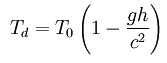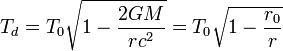## temporary dilations

According to the theory of general relativity, accelerating systems would also experience time dilation similar to what occurs in a gravitational field. Similarly, in reference systems such as a carousel or a Ferris wheel, time dilation will appear as an effect of your spins.

#### Examples of Gravitational Time Dilation

• In an accelerated box, the equation with respect to an arbitrary observer would be:Whereis the elapsed time measured by the accelerated observer,is the acceleration of the box measured by the base observer, andis the vertical distance between observers.

• In a rotating disk when the observer is positioned in the center of the disk and rotating with the:Whereis the distance to the center of the disk,is the angular velocity of the disk.

• under the solar system, we would have:Whereis the proper time for events A and B for the slow observer within the gravitational field andis the proper time of the fast observer,is the gravitational constant,is the apparent mass of the object that creates the gravitational field,is the radial coordinate of the observer,is the speed of light and#### experimental confirmation

It should be noted that time dilation has been able to be measured experimentally using atomic clocks in airplanes., where the clocks in the planes were slightly ahead of those on the ground.

We have a special category of Physics books in our geeks gifts online shop,

so you can understand in depth the nature of the Time travel.